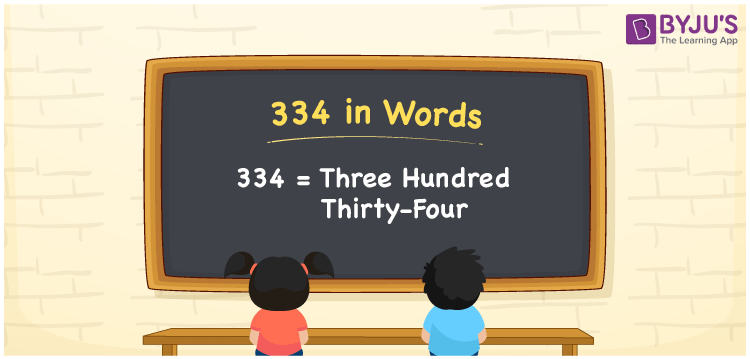# 334 in Words

We can write 334 in words as Three hundred thirty-four. If you bought a special pizza for Rs. 334, then you can say, “I bought a special pizza for Three hundred thirty-four rupees”. Here, Three hundred thirty-four is the number name of the cardinal number 334. In this article, you will learn how to convert 334 into words and some facts about 334.

 334 in words Three hundred thirty-four Three hundred thirty-four in Numbers 334

## 334 in English Words

We generally use the English alphabet to express numbers in words. So, we spell 334 in English words as “Three hundred thirty-four”.## How to Write 334 in Words?

Let’s learn how to write the number 334 in words using a place value chart with three columns since it has three digits. The palace values for all the digits of 334 are given below.

 Hundreds Tens Ones 3 3 4

Here, ones = 4, tens = 3, hundreds = 3

By expanding these digits with respect to the place values, we get;

3 × Hundred + 3 × Ten + 4 × One

= 3 × 100 + 3 × 10 + 4 × 1

= 300 + 30 + 4

= Three hundred + Thirty + Four

= Three hundred thirty-four

Therefore, 334 in words is written as Three hundred thirty-four.

334 is a natural number that precedes 335 and succeeds 333.

334 in words – Three hundred thirty-four

Is 334 an odd number? – No

Is 334 an even number? – Yes

Is 334 a prime number? – No

Is 334 a composite number? – Yes

Is 334 a perfect square number? – No

Is 334 a perfect cube number? – No

## Frequently Asked Questions on 334 in Words

Q1

### How do you write 334 in words?

We can write 334 in words as Three hundred thirty-four.
Q2

### Write the number 334.08 in words.

The word form of the decimal number 334.08 is “Three hundred thirty-four point zero eight”.
Q3

### Express the value of 334 + 101 in words.

334 + 101 = 435 Therefore, the value of 334 + 101, i.e., 435 can be expressed in words as Four hundred thirty-five.